Moments - Measures of Dispersion, Business Mathematics & Statistics

# Moments - Measures of Dispersion, Business Mathematics & Statistics - Business Mathematics and Statistics - B Com

• Moments

A moment designates the power to which deviation are raised before averaging them.

Methods of Standard Deviation
1. Moments about Mean or Central Moments
2. Moments about Origin or Zero
3. Moments about Provisional Mean or Arbitrary Value (Non Central Moment)

1. Moments about Mean or Central Moments

For Ungrouped Data​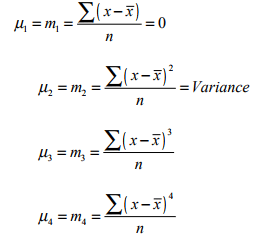For Grouped Data​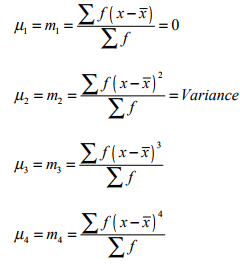2. Moments about Origin or Zero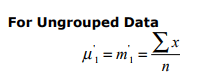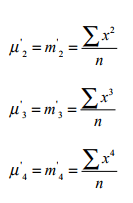For Grouped Data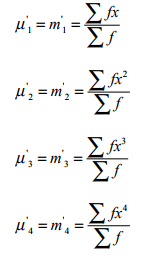3. Moments about Provisional Mean or Arbitrary Value (Non Central Moment)
4. Methods of Standard Deviation

i. Direct Method
ii. Short Cut Method
iii. Coding Method or Step-Deviation Method

i. Direct Method For Ungrouped Data

For Ungrouped Data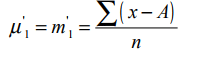Where A is constant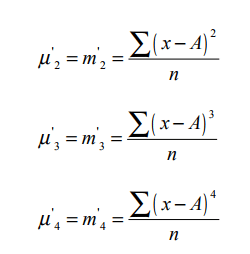For Grouped Data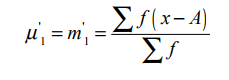Where A is constant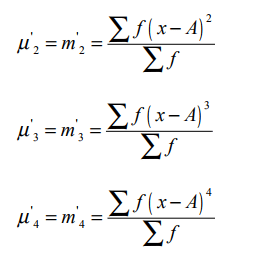ii. Short Cut Method
For Ungrouped Data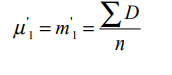Where D= X - A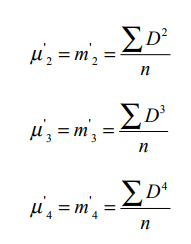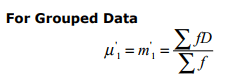Where D= X - A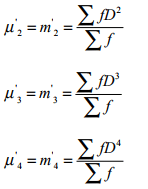iii. Coding Method or Step Deviation Method
For Ungrouped Data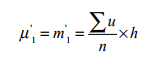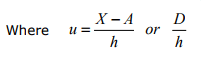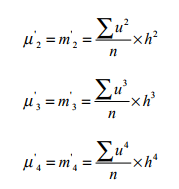For Grouped Data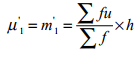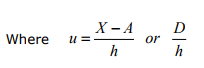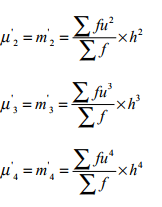• Relation Between Central moments in Terms of Non Central Moments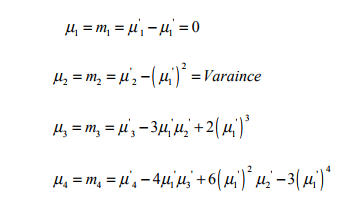• Moments – Ration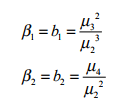• Sheppard’s Correction for Moments of Group Data​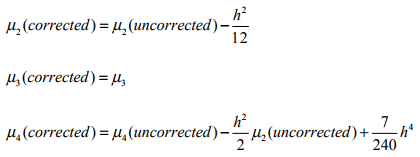Charliers Check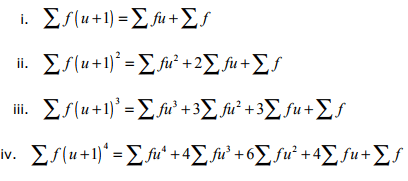• Symmetry
In a symmetrical distribution a deviation below the mean exactly equals the corresponding deviation above the mean. It is called symmetry. For symmetrical distribution the following relations hold.

Mean = Median = Mode
Q3 - Median = Median - Q1
u3 = m3 = 0
β1 = b1 = 0

• Skewness
Skewness is the lack of symmetry in a distribution around some central value i.e. means Median or Mode. It is the degree of asymmetry.

Mean ≠ Median ≠ Mode
Q3 - Median ≠ Median - Q1
u3  = m3 ≠ 0
β1 = b1 ≠ 0 There are two types of Skewness

1. Positive Skewness
If the frequency curve has a longer tail to right, the distribution is said to be positively skewed.

2. Negative Skewness
If the frequency curve has a longer tail to left, the distribution is said to negatively skewed.

Coefficient of Skewness (SK)

Karl Pearson’s Coefficient of Skewness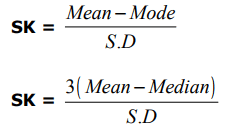Bowly’s Quartile Coefficient of Skewness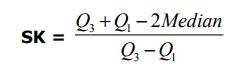Moment Coefficient of Skewness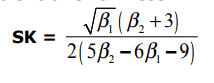Kurtosis

Moment coefficient β2 is an important measure of kurtosis. These measures define as: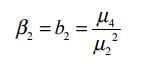The moment coefficient β2 is a pure numbers and independent of the origin and unit of measurement.

If β2 > 3 distribution is Leptokurtic
If β2 = 3 distribution is Normal or Mesokurtic
If β< 3 distribution is Platy Kurtic

Or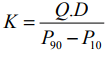For Normal distribution, K = 0.263

The document Moments - Measures of Dispersion, Business Mathematics & Statistics | Business Mathematics and Statistics - B Com is a part of the B Com Course Business Mathematics and Statistics.
All you need of B Com at this link: B Com

115 videos|142 docs

## FAQs on Moments - Measures of Dispersion, Business Mathematics & Statistics - Business Mathematics and Statistics - B Com

 1. What are measures of dispersion in statistics?Ans. Measures of dispersion in statistics are numerical values that measure the spread or variability of a dataset. They provide information about the extent to which the data points deviate from the central tendency. Examples of measures of dispersion include range, variance, standard deviation, and coefficient of variation.
 2. How is range calculated as a measure of dispersion?Ans. Range is a simple measure of dispersion that represents the difference between the highest and lowest values in a dataset. To calculate the range, subtract the lowest value from the highest value. For example, if the highest value in a dataset is 50 and the lowest value is 10, the range would be 50 - 10 = 40.
 3. What is the difference between variance and standard deviation?Ans. Variance and standard deviation are both measures of dispersion, but they represent it in different ways. Variance measures the average squared deviation of each data point from the mean, while standard deviation measures the average deviation of each data point from the mean. To calculate the standard deviation, take the square root of the variance.
 4. How is the coefficient of variation useful in comparing datasets?Ans. The coefficient of variation (CV) is a measure of relative variability and is useful in comparing datasets with different units or scales. It is calculated by dividing the standard deviation by the mean and multiplying by 100 to express it as a percentage. A lower CV indicates less variability relative to the mean, while a higher CV indicates greater variability.
 5. Why are measures of dispersion important in business and statistics?Ans. Measures of dispersion are important in business and statistics because they provide valuable insights about the variability and distribution of data. They help identify outliers, assess the risk associated with certain variables, compare different datasets, and make informed decisions. By understanding the spread of data, businesses and statisticians can better analyze trends, predict outcomes, and mitigate risks.

115 videos|142 docsExplore Courses for B Com examSignup to see your scores go up within 7 days! Learn & Practice with 1000+ FREE Notes, Videos & Tests.
10M+ students study on EduRev
Track your progress, build streaks, highlight & save important lessons and more!
Related Searches

,

,

,

,

,

,

,

,

,

,

,

,

,

,

,

,

,

,

,

,

,

,

,

,

;